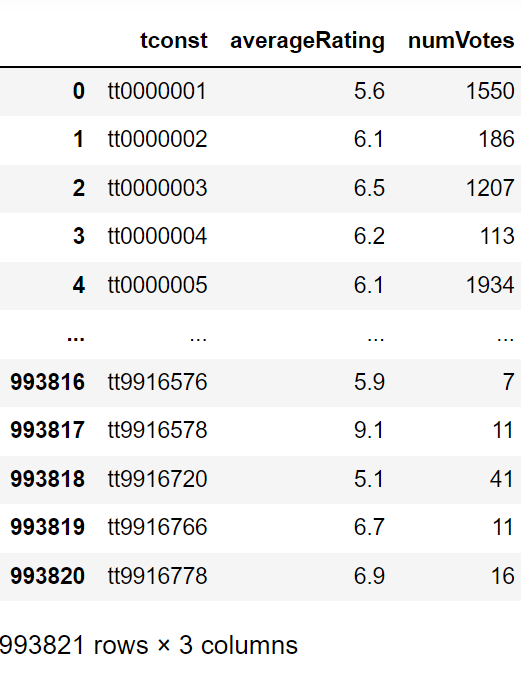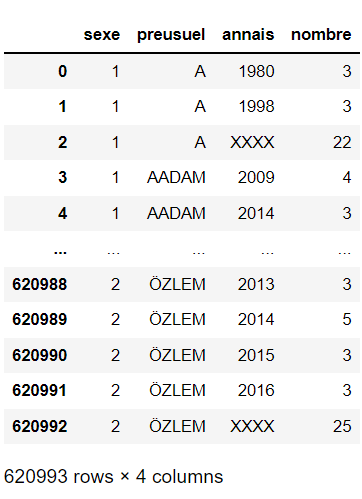How to load a TSV file into a Pandas DataFrame?

• Last Updated : 18 Oct, 2021

The idea is extremely simple we only have to first import all the required libraries and then load the data set by the use of read_csv() method. To this method simply the path of the dataframe is passed. This is enough to get the job done.

Syntax :

Given below are some implementations for the same.

Dataset Used:  data.tsv

Python3

 import pandas as pd  # Data.tsv is stored locally in the # same directory as of this python filedf = pd.read_csv('data.tsv',sep = '\t') df

Output:Dataset used: babynames.tsv

Python3

 import pandas as pd  df = pd.read_csv('babynames.tsv', sep = '\t')df

Output:My Personal Notes arrow_drop_up